Subscribe

# GenericValue Variables

The GenericValue variable is a type of variable that can store any kind of data, including text, numbers, dates, and arrays, and is particular to UiPath Studio.

GenericValue variables are automatically converted to other types, in order to perform certain actions. However, it is important to use these types of variables carefully, as their conversion may not always be the correct one for your project.

UiPath Studio has an automatic conversion mechanism of GenericValue variables, which you can guide towards the desired outcome by carefully defining their expressions. Take into account that the first element in your expression is used as a guideline for what operation Studio performs. For example, when you try to add two GenericValue variables, if the first one in the expression is defined as a String, the result is the concatenation of the two. If it is defined as an Integer, the result is their sum.

# Supported .NET Methods

Name/SyntaxDescription
CompareTo
`Function GenericValue.CompareTo(other As GenericValue) As Integer`

`Function GenericValue.CompareTo(obj As Object) As Integer`
: https://msdn.microsoft.com/en-us/library/system.string.compareto(v=vs.110).aspx
Contains
`Function GenericValue.Contains(other As String) As Boolean`
: https://msdn.microsoft.com/en-us/library/dy85x1sa(v=vs.110).aspx

Used to check whether a string variable contains a certain substring or not. For example, if you want to check if a sentence contains a specific word, the expression should be `[SentenceVariable].Contains("term")`, where `[SentenceVariable]` is the GenericValue variable containing the sentence and `"term"` is the word to be searched for.

Note: When using this method with a GenericValue variable of data type Int, Float or Boolean, the data is automatically converted to String and handled accordingly.
Equals
`Function GenericValue.Equals(other As GenericValue) As Boolean`

`Function GenericValue.Equals(obj As Object) As Boolean`
: https://msdn.microsoft.com/en-us/library/system.string.equals(v=vs.110).aspx
GetHashCode
`Function GenericValue.GetHashCode() As Integer`
: https://msdn.microsoft.com/en-us/library/system.string.format(v=vs.110).aspx
GetType
`Function Object.GetType() As Type`
: https://msdn.microsoft.com/en-us/library/system.object.gettype(v=vs.110).aspx
GetTypeCode
`Function GenericValue.GetTypeCode() As TypeCode`
: https://msdn.microsoft.com/en-us/library/system.string.gettypecode(v=vs.110).aspx
IndexOf
`Function GenericValue.IndexOf(value As String) As Integer`

`Function GenericValue.IndexOf(value As String, comparisonType As StringComparison) As Integer`

`Function GenericValue.IndexOf(value As String, startIndex As Integer) As Integer`

`Function GenericValue.IndexOf(value As String, startIndex As Integer, comparisonType As StringComparison) As Integer`
: https://msdn.microsoft.com/en-us/library/system.string.indexof(v=vs.110).aspx

Used to return the index of a substring contained inside a string variable. For example, if you want to find the index of the word "locate" inside the sentence "Find 'locate' in this sentence", the expression should be `[SentenceVariable].IndexOf("locate")`, where `[SentenceVariable]` is the GenericValue variable containing the sentence and `"locate"` is the term to be searched for.

Note: When using this method with a GenericValue variable of data type Int, Float or Boolean, the data is automatically converted to String and handled accordingly.
Length
`Function GenericValue.Lenght() As Integer`
: https://msdn.microsoft.com/en-us/library/system.string.length(v=vs.110).aspx

Used to return the number of characters in a string variable. For example, if you want to find out how many letters a word has, the expression should be `[WordVariable].Length`, where `[WordVariable]` is the GenericValue variable containing the word.

Note: When using this method with a GenericValue variable of data type Int, Float or Boolean, the data is automatically converted to String and handled accordingly.
Replace
`Function GenericValue.Replace(oldValue As String, new value As String) As String`
: https://msdn.microsoft.com/en-us/library/system.string.replace(v=vs.110).aspx

Used to replace data contained inside a string variable. For example, if you want to change a local file path `C:\ExampleFolder\Main.xaml` into the corresponding server file path `C:/ExampleFolder/Main.xaml`, the expression should be `[PathVariable].Replace("\","/")` where `[PathVariable]` is the GenericValue variable containing the file path, `"\"` is the character to be replaced and `"/"` is the character used as replacement.

Note: When using this method with a GenericValue variable of data type Int, Float or Boolean, the data is automatically converted to String and handled accordingly.
Split
`Function GenericValue.Split(ParamArray separator As Char()) As String()`

`Function GenericValue.Split(ParamArray separator As String()) As String()`

`Function GenericValue.Split(separator As Char(), options As StringSplitOptions) As String()`

`Function GenericValue.Split(separator As String(), options As StringSplitOptions) As String()`
: https://msdn.microsoft.com/en-us/library/system.string.split(v=vs.110).aspx

Used to return individual components from a string variable. For example, if you want to extract the year from a MM.DD.YYYY date format, the expression should be `[DateVariable].Split(".".ToCharArray)(2)`, where `[DateVariable]` is the GenericValue variable containing the date, `"."` is the character used as a separator, `.ToCharArray` is a method that creates an array with the elements delimited by the separator and `(2)` represents the index of the element to be returned, in our case, the year.

Note: When using this method with a GenericValue variable of data type Int, Float or Boolean, the data is automatically converted to String and handled accordingly.
Substring
`Function GenericValue.Substring(startIndex As Integer) As String`

`Function GenericValue.Substring(startIndex As Integer, length As Integer) As String`
: https://msdn.microsoft.com/en-us/library/system.string.substring(v=vs.110).aspx

Used to return a substring contained in a string variable. For example, if you want to extract a word from the "There are 100 machines available" sentence, the expression should be `[SentenceVariable].Substring(10,3)`, where `[SentenceVariable]` is the GenericValue variable containing the sentence, 10 is the index of the first character to be returned, and 3 is the length of the substring starting from the first character. In this example, the resulted substring would be "100".

Note: When using this method with a GenericValue variable of data type Int, Float or Boolean, the data is automatically converted to String and handled accordingly.
ToBoolean
`Function GenericValue.ToBoolean(provider As IFormatProvider) As Boolean`
: https://msdn.microsoft.com/en-us/library/bb346937(v=vs.110).aspx
ToByte
`Function GenericValue.ToByte(provider As IFormatProvider) As Byte`
: https://msdn.microsoft.com/en-us/library/bb355898(v=vs.110).aspx
ToChar
`Function GenericValue.ToChar(provider As IFormatProvider) As Char`
: https://msdn.microsoft.com/en-us/library/bb335877(v=vs.110).aspx
ToDecimal
`Function GenericValue.ToDecimal(provider As IFormatProvider) As Decimal`

`Function GenericValue.ToDecimal(format As NumberStyles) As Decimal`
: https://msdn.microsoft.com/en-us/library/bb359959(v=vs.110).aspx
ToDouble
`Function GenericValue.ToDouble(provider As IFormatProvider) As Double`

`Function GenericValue.ToDouble(format As NumberStyles) As Double`
: https://msdn.microsoft.com/en-us/library/bb154906(v=vs.110).aspx
ToInt
`Function GenericValue.ToInt(culture As IFormatProvider) As Integer?`

`Function GenericValue.ToInt() As Integer?`
Used to convert a specified value to a nullable integer.

Note: When using this method with a GenericValue variable of data type Boolean, the "True" and "False" values are converted to 1 and 0, respectively.
ToInt16
`Function GenericValue.ToInt16(provider As IFormatProvider) As Short`
: https://msdn.microsoft.com/en-us/library/bb293091(v=vs.110).aspx

Note: When using this method with a GenericValue variable of data type Boolean, the "True" and "False" values are converted to 1 and 0, respectively.
ToInt32
`Function GenericValue.ToInt32(provider As IFormatProvider) As Integer`
: https://msdn.microsoft.com/en-us/library/bb300452(v=vs.110).aspx

Note: When using this method with a GenericValue variable of data type Boolean, the "True" and "False" values are converted to 1 and 0, respectively.
ToInt64
`Function GenericValue.ToInt64(provider As IFormatProvider) As Long`
: https://msdn.microsoft.com/en-us/library/bb359566(v=vs.110).aspx

Note: When using this method with a GenericValue variable of data type Boolean, the "True" and "False" values are converted to 1 and 0, respectively.
ToLower
`Function GenericValue.ToLower() As String`
: https://msdn.microsoft.com/en-us/library/system.string.tolower(v=vs.110).aspx

Note: When using this method with a GenericValue variable of data type Int, Float or Boolean, the data is automatically converted to String and handled accordingly.
ToLowerInvariant
`Function GenericValue.ToLowerInvariant() As String`
: https://msdn.microsoft.com/en-us/library/system.string.tolowerinvariant(v=vs.110).aspx

Note: When using this method with a GenericValue variable of data type Int, Float or Boolean, the data is automatically converted to String and handled accordingly.
ToSByte
`Function GenericValue.ToSByte(provider As IFormatProvider) As SByte`
: https://msdn.microsoft.com/en-us/library/bb342232(v=vs.110).aspx
ToSingle
`Function GenericValue.ToSingle(provider As IFormatProvider) As Single`
: https://msdn.microsoft.com/en-us/library/bb360375(v=vs.110).aspx
ToString
`Function GenericValue.ToString(formatProvider As IFormatProvider) As String`

`Function GenericValue.ToString(format As String, formatProvider As IFormatProvider) As String`

`Function GenericValue.ToString() As String`
: https://msdn.microsoft.com/en-us/library/system.string.tostring(v=vs.110).aspx
ToType
`Function GenericValue.ToType(conversionType As Type, provider As IFormatProvider) As Object`
: https://msdn.microsoft.com/en-us/library/bb358540(v=vs.110).aspx
ToUInt16
`Function GenericValue.ToUInt16(provider As IFormatProvider) As UShort`
: https://msdn.microsoft.com/en-us/library/bb337265(v=vs.110).aspx

Note: When using this method with a GenericValue variable of data type Boolean, the "True" and "False" values are converted to 1 and 0, respectively.
ToUInt32
`Function GenericValue.ToUInt32(provider As IFormatProvider) As UInteger`
: https://msdn.microsoft.com/en-us/library/bb357762(v=vs.110).aspx

Note: When using this method with a GenericValue variable of data type Boolean, the "True" and "False" values are converted to 1 and 0, respectively.
ToUInt64
`Function GenericValue.ToUInt64(provider As IFormatProvider) As Ulong`
: https://msdn.microsoft.com/en-us/library/bb155099(v=vs.110).aspx

Note: When using this method with a GenericValue variable of data type Boolean, the "True" and "False" values are converted to 1 and 0, respectively.
ToUpper
`Function GenericValue.ToUpper() As String`
: https://msdn.microsoft.com/en-us/library/system.string.toupper(v=vs.110).aspx

Note: When using this method with a GenericValue variable of data type Int, Float or Boolean, the data is automatically converted to String and handled accordingly.
ToUpperInvariant
`Function GenericValue.ToUpperInvariant() As String`
: https://msdn.microsoft.com/en-us/library/system.string.toupperinvariant(v=vs.110).aspx

Note: When using this method with a GenericValue variable of data type Int, Float or Boolean, the data is automatically converted to String and handled accordingly.
Trim
`Function GenericValue.Trim(ParamArray trimChars As Char()) As String`

`Function GenericValue.Trim() As String`
: https://msdn.microsoft.com/en-us/library/system.string.trim(v=vs.110).aspx

Note: When using this method with a GenericValue variable of data type Int, Float or Boolean, the data is automatically converted to String and handled accordingly.
TrimEnd
`Function GenericValue.TrimEnd(ParamArray trimChars As Char()) As String`
: https://msdn.microsoft.com/en-us/library/system.string.trimend(v=vs.110).aspx

Note: When using this method with a GenericValue variable of data type Int, Float or Boolean, the data is automatically converted to String and handled accordingly.
TrimStart
`Function GenericValue.TrimStart(ParamArray trimChars As Char()) As String`
: https://msdn.microsoft.com/en-us/library/system.string.trimstart(v=vs.110).aspx

Note: When using this method with a GenericValue variable of data type Int, Float or Boolean, the data is automatically converted to String and handled accordingly.

When using Ctrl + K, Ctrl + M, or Ctrl + Shift + M directly in the Format Value activity body, the created variable or argument is of type GenericValue. For other activities, the created type is the same as the required type of the activity.

# Example of Using a GenericValue Variable

To demonstrate how a GenericValue variable works, let us create an automation that performs different operations whose results depend on the way we define their expressions. We create two GenericValue variables of different data types and display the results in the Output panel.

1. Create a new blank project.
2. Create three GenericValue variables: `Int`, `Str`, and `Result`.
3. In the Default column for the `Int` variable, type 12, and for the `Str` variable, type "34". The first variable is interpreted as an integer, while the second one is interpreted as a string.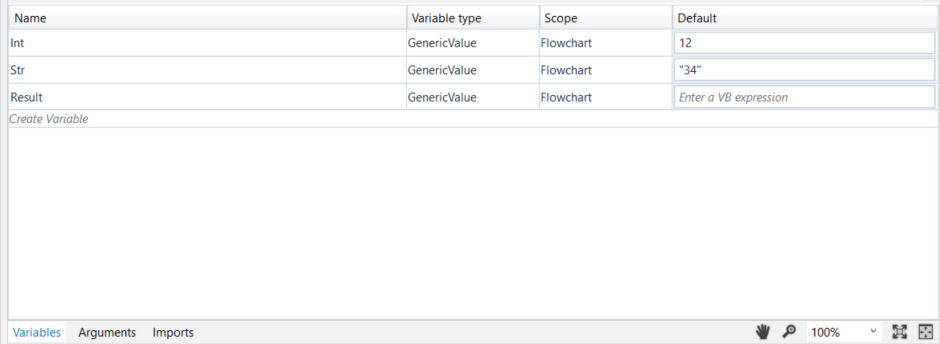1. Add an Assign activity to the Designer panel and connect it to the Start node.
2. In the Properties panel, in the To field, enter the `Result` variable.
3. In the Value field, type `Int` + `Str`.
4. Add a Write Line activity and connect it to the Assign one.
5. In the Properties panel, in the Text field, enter the `Result` variable.
The project should look as in the following screenshot.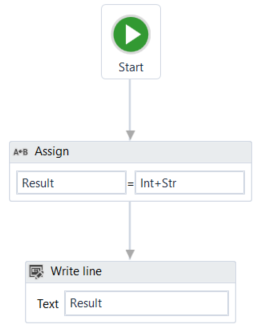1. Press F5 to execute your automation. Note that, in the Output panel, the sum of the two numbers is displayed.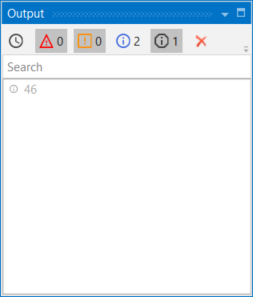1. Go back to the previously added Assign activity and change the Value field to `Str` + `Int`, to reverse the order of the variables. The project should look as in the following screenshot.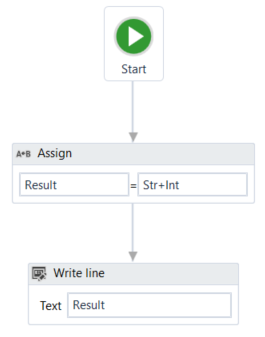1. Press F5 to execute your automation. Note that, in the Output panel, the concatenation of the two numbers is displayed.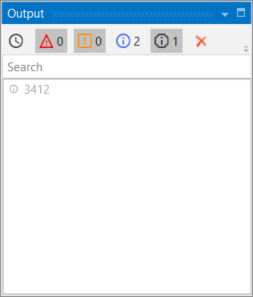This means that UiPath Studio takes the first element defined in your expression as a guideline for what operation to perform. If the first element in your expression is an integer or a GenericValue variable filled in as integer, UiPath Studio will perform the sum of the elements.
If the first element in your expression is a string or a GenericValue variable filled in as string, UiPath Studio will perform the concatenation of the elements.Updated 2 years ago